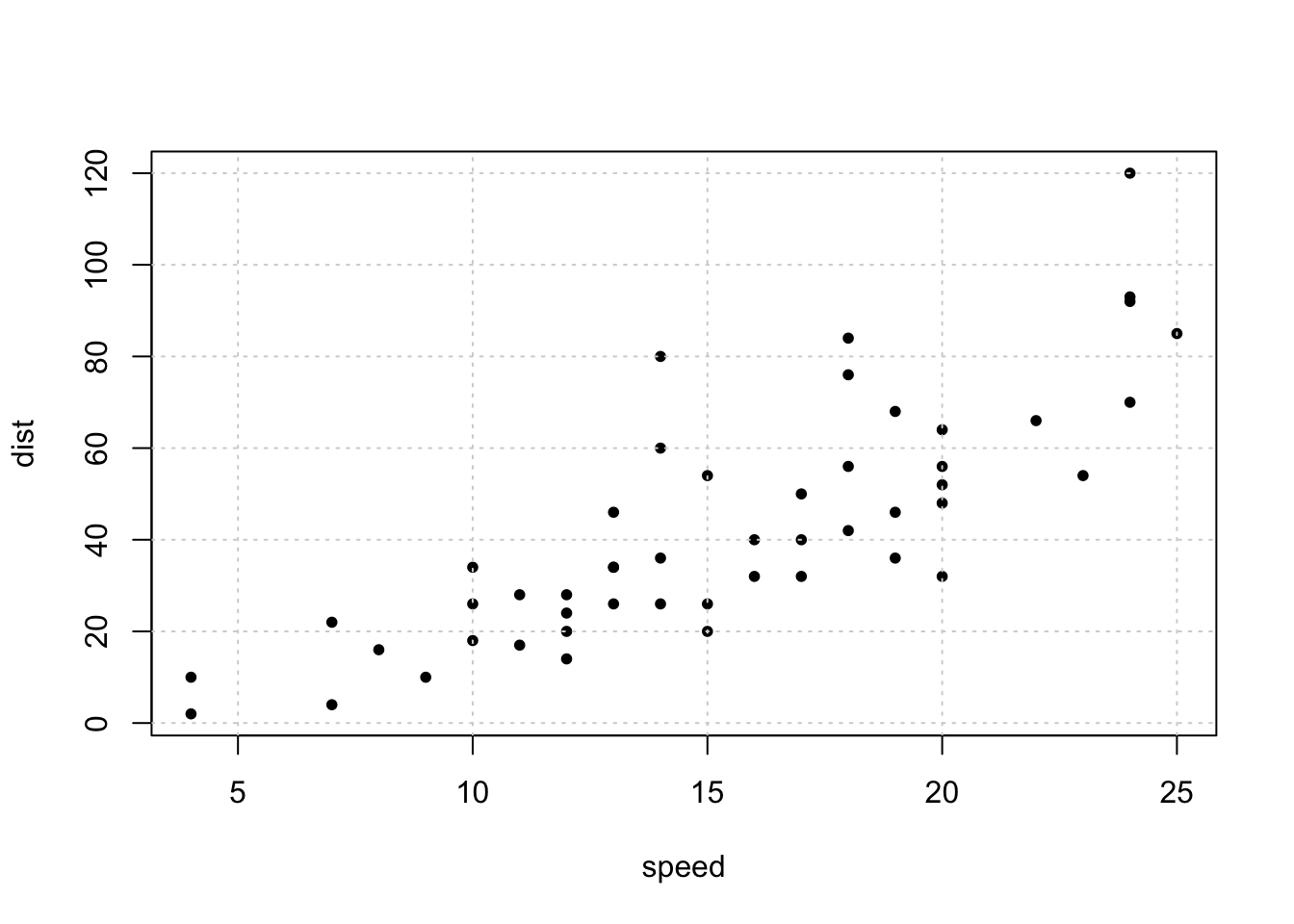Goal: After completing this lab, you should be able to…

• Use the `lm()` function in `R` to fit regression models.
• Plot fitting SLR models.
• Predict new observations using SLR models.

In this lab we will use, but not focus on…

• `R` Markdown. This document will serve as a template. It is pre-formatted and already contains chunks that you need to complete.

• You may use this document as a template. You do not need to remove directions. Chunks that require your input have a comment indicating to do so.
• The following readings may be very useful:

# Exercise 0 - Cars

In class we looked at the (boring) `cars` dataset. Use `?cars` to learn more about this dataset. (For example, the year that it was gathered.)

``head(cars)``
``````plot(dist ~ speed, data = cars, pch = 20)
grid()``````Our purpose with this dataset was to fit a line that summarized the data. We did this with the `lm()` function in `R`.

``cars_mod = lm(dist ~ speed, data = cars)``

Using the `summary()` function on the result of the `lm()` function produced some useful output, including the slope and intercept of the line that we fit.

``summary(cars_mod)``
``````##
## Call:
## lm(formula = dist ~ speed, data = cars)
##
## Residuals:
##     Min      1Q  Median      3Q     Max
## -29.069  -9.525  -2.272   9.215  43.201
##
## Coefficients:
##             Estimate Std. Error t value Pr(>|t|)
## (Intercept) -17.5791     6.7584  -2.601   0.0123 *
## speed         3.9324     0.4155   9.464 1.49e-12 ***
## ---
## Signif. codes:  0 '***' 0.001 '**' 0.01 '*' 0.05 '.' 0.1 ' ' 1
##
## Residual standard error: 15.38 on 48 degrees of freedom
## Multiple R-squared:  0.6511, Adjusted R-squared:  0.6438
## F-statistic: 89.57 on 1 and 48 DF,  p-value: 1.49e-12``````

We could use the `abline()` function to add this line to a plot.

``````plot(dist ~ speed, data = cars, pch = 20)
grid()
abline(cars_mod, col = "red")``````